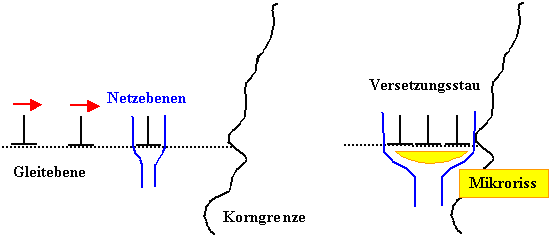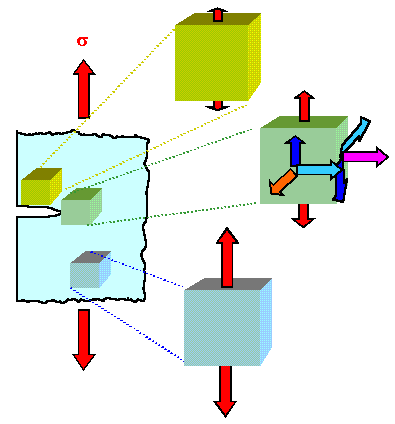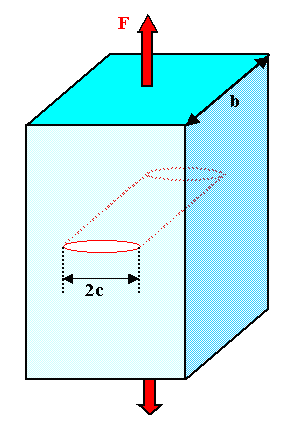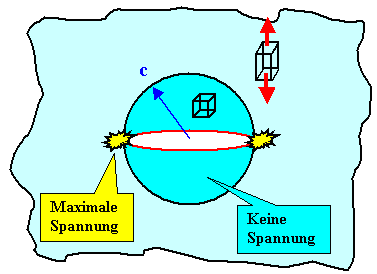# Why is some material not perfectly elastic?What are microcracks? First of all, small cavities or "voids", especially if they are more two-dimensional. But there is a lot more:Literally small ones that lead from the surface into the material. Any mechanically processed surface will have such microcracks, even if they are not visible to the naked eye or the light microscope., e.g. between grains, especially between sintered grains of a ceramic, or on precipitates that do not quite fit into the grid. Only a few like these microcracks nm be extended; but that is enough to influence the fracture behavior.e.g. at a grain boundary. In the extreme case it looks like this:Any tension drives the drawn step dislocations to the right (left part of the picture), where they meet an impenetrable barrier, e.g. a grain boundary or a precipitate. The Relocation jam as a result is shown on the right; the area below the crushed dislocations is "practically" a small crack.With this one can generally say: Every "thinned" zone in the crystal can be understood as a microcrack - and all of this then applies accordingly to amorphous materials as well. The crucial insight now is:. There are reasons for this:1.. The picture on the right shows a stress-free microcrack in the unloaded state (only some material has been removed from the surface). If we apply a uniaxial stress from the outside (i.e. we do a tensile test), we also only find a uniaxial stress state on differential unit cubes (far away from the crack).On a unit cube close to the microcrack, however, the tensions are different - directly on the crack there is simply no material that can stand up to the pull from above or below. We have to relieve or build up tension so that the cube stays at rest; the picture shows this schematically. The stresses are lower close to the crack surface and greater at the crack tip.This means that after local transformation on the main axes, the (fracture-causing) normal stresses can now be higher than the external stress; especially on sharp "edges". This results in local breakage at the microcrack when there is tension than in the volume.2. Many microcracks in the general definition (e.g. the dislocation jam) already have high built-in stress; usually areas with both tensile and compressive stresses.At the micro-crack, the built-in stress and the applied external stress are superimposed in such a way that the normal stresses add up. Again the local stresses are higher than the external stress; local break follows global break.Both effects can of course overlap, but in the end we will practically always be able to assume that a local break at the microcrack occurs long before the global break.The situation is quite complex; it does not look as if it would be easily possible to develop a simple break criterion for breaks in microcracks of all kinds. It doesn't just look like it either - it is!- We are not that keen to find out whether a break occurs at any micro-crack, i.e. the crack enlarges. What we really want to know is whether!So the important question is whether at least the local microcracks can be found - until it encompasses the entire sample. And for that, for them Spread of microcracks, nice enough to find simple criteria.Well run! We will see.We consider an (idealized) microcrack in an otherwise perfect crystal in the geometry shown below.We just have to figure out whether it is going to enlarge the rift. In other words: Takes the elastic energy stored in the crystal with the crack P.* as a function of the microcrack area 2c b now and then, if we apply a voltage.The criterion for crack propagation is then whether P.*Crack gets bigger or smaller. In formulas:
dP.*Crack d (c · b) i.e. the crack will spread i.e. the crack will widenHow big is the elastic energy stored in the crack-free crystal? In the crystal it is given for a linear course of the stress-strain curve by the toughness introduced earlier, i.e..The tension in the area under consideration is identical everywhere with the externally applied tension, and the elongations (in the tensile direction) result from.Far away from the microcrack, its influence on the stress and strain field will be minimal and therefore negligible. The above formula then applies to this part of the crystal.Around the crack, however, the stresses and strains will be than in the volume of the crystal, and thus also the stored elastic energy.How exactly the stresses and strains appear around the microcrack, and thus the stored elastic energy, is a difficult problem of elasticity theory; the solutions are of course dependent on the exact type of microcrack. But we don't want to burden ourselves with this and do one:We assume that in one area around the micro-crack the stresses and strains are simple.Let's look at what that means:No stresses and strains can act directly on the surface of the microcrack - the atoms close to the surface experience no forces and are always at an equilibrium distance from their neighbors (let's ignore the "corners").This seems to be in contradiction to the above statement of increased stresses on a microcrack, but the excessive stress only occurs on a massive scale at "sharp" corners.In principle, we can expect that in a volume corresponding to the size of the microcrack, the stresses and strains are overall smaller than in the volume. Our approximation takes this into account; but we cannot really estimate how good it is.However, it is relatively easy for us to arrive at a formula that, despite being approximated, has some significance.So elastic energy is stored in the cylinder volume, or it becomes energy P.Crack released if we now introduce a crack in a given volume.The energy is thus:
P.Crack  =  2
2E.
·   · c2 · bThe second term is simply the volume of the cylinder.With the crack area A. = · [2c ·b] (the factor takes into account that there are crack surfaces)
P.Crack  =  2
2E.
·   · c · A.
4This elastic energy represents the P.If = · A. = 4 · c · b opposite.We can use it to reformulate the rupture criterion from above: rupture will occur if the elastic energy is greater than the surface energy when the micro-crack is enlarged.The criterion for Crack growth so is in formulas
dP.If
dA.
<  dP.Crack
dA.With that we have
d
dA.
( · A.)  <  d
dA.
2 · · c · A.
8E.

<  2 · · c
8E.As a final result, we getBreak occurs forIt's a simple and useful formula. In particular, it says that a microcrack in the material if the external tensile stress is greater than a critical stress that depends on the!How good is the formula? Finally, we used a pretty radical approximation.The answer is: - within the idealized state. More accurate (and much more complex) calculations yield just one factor 2 Difference; we obtain:
>  2E. ·
· c
1/2In particular, the functional dependence of the breaking stress on the and the is unchanged.Let's compare the stress at break in the presence of microcracks (we'll call them Crack), with the maximum theoretical breaking strengthMax, we receive
Max
Crack
=   · c
r0The maximum real breaking stress of a material is therefore by the factor · c/r0 than the theoretical breaking stress. It is c the dimension of the.The formula shows in particular: Even the smallest micro-cracks in the area of ​​fewer lattice constants r0, i.e. in the nanometer range, significantly reduce the breaking strength.We also understand why the fragments of an already broken sample now tend to break only at higher stresses: The remaining microcracks can only be smaller than the microcrack that led to the first break.What does the experiment say about our formula? To the independent predictions
Crack    ½

Crack   (1 / c)½While (the not very easy to measure) dependence on the crack size c is generally quite well fulfilled; this applies to the proportionality to the root of the surface energy.The surface energy resulting from fracture experiments often tends to be considerably greater than the true surface energy.This means that there is energy in the (enlarged) crack than can be "accommodated" in the new surfaces. In practice this is a good thing - it increases the real breaking strength.The reason for this observation is usually that something is happening at the tip of the crack - even in the case of actually brittle materials! Dislocations are generated and moved - and this requires energy. This is the energy found in the experiment, which simulates an increased surface energy.We are ending with that - although many questions remain unanswered and there is still a lot to be said. Hopefully it has become clear why engineering fracture mechanics - e.g. the prediction of which component will break under which load and when - is one of the most difficult problems in structural materials science (note the previously unaddressed development of the fracture stress, i.e. the aging behavior of the component with regard to brittle fracture ).And let's not forget: the structural integrity of a product is the basic prerequisite for its functioning. Major technical disasters are often due to breaks (not necessarily just breaks).If you would like to know more: In an "advanced" module, a few points are deepened.

© H. Föll (MaWi 1 script)